# 测试数据构造秘技（1） ——专属数据引用数据分离

Csv1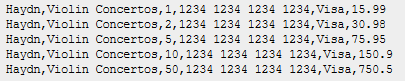Csv2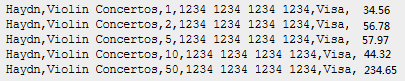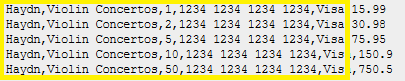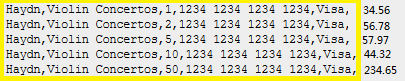• 应用程序引用数据 (Application reference data) 是测试无关数据，但它们是应用程序启动所必需的，这些数据往往是指一些基表和基础数据；
• 测试引用数据（Test reference data）是那些和测试相关，但是对测试行为没有多大影响的数据，在下面两个例子中，黄色就是测试引用数据• 测试专属数据（Test specific data）：真正影响测试行为的特征数据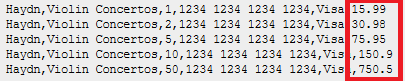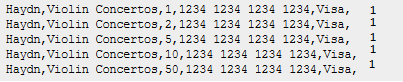UPDATE transactions SET amount = 15.99 WHERE id = 1;
UPDATE transactions SET amount = 30.98 WHERE id = 2;
UPDATE transactions SET amount = 75.95 WHERE id = 5;
UPDATE transactions SET amount = 150.9 WHERE id = 10;UPDATE transactions SET amount = 750.5 WHERE id = 50;UPDATE transactions SET amount = 34.56 WHERE id = 1;
UPDATE transactions SET amount = 56.78 WHERE id = 2;
UPDATE transactions SET amount = 57.97 WHERE id = 5;
UPDATE transactions SET amount = 44.32 WHERE id = 10;
UPDATE transactions SET amount = 234.65 WHERE id = 50;

• 测试案例可维护性：上面这些案例中，测试引用数据由于使用了 INSERT 语句，它其实会受到数据库表结构变化的影响，而测试专属数据准备由于使用 UPDATE 语句，不会受到数据库表结构变化的影响。我们通过统一测试引用数据准备程序，将这种变化的冲击大大降低，未来数据表结构变更，我们只需修改统一的测试引用数据准备程序而无需修改每一个案例，这其实暗合了 DRY 原则（Don’t repeat yourself）。
• 测试案例可读性：由于我们将测试引用数据准备从独立出来了，只要看测试案例本身，就可以明确地看到测试专属数据，被测行为和结果验证，让案例可读性大大提升。

XML1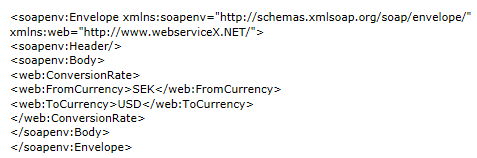XML2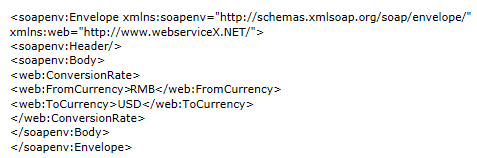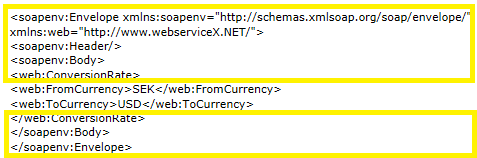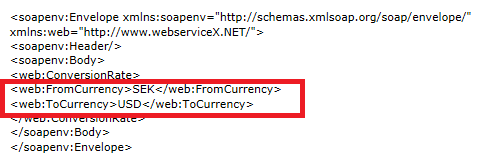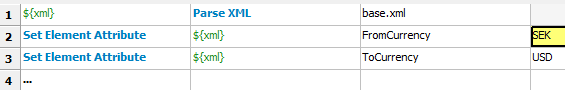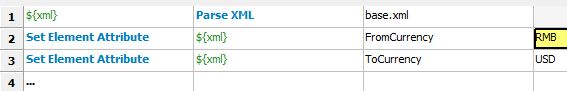Base.xml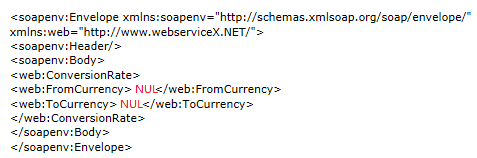## 作者简介

wuqiong@agilean.cn